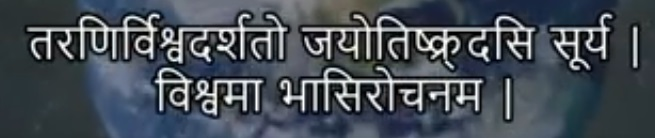# Speed of Light: Rigveda vs Modern ScienceThe above verse is given from verse number 50 from Rigveda book number 1 and its literal translation is given as follows

Light travels 2202 yojanas in one-half nimisha

So let us discuss this in detail

Nimisha is a unit of time used ancient India which is having the following conversions

1 kastha = 15 nimishas

1 kala = 30 kasthas

1 mahurta = 30.3 kalas

1 diva ratri = 30 mahurtas

and

1 diva ratri = 1 day – night = 24 hours of modern time unit

if you do a little bit of maths you can see that it comes to

1 nimisha = 0.2112 seconds of modern time

so one-half nimisha = 0.1056 seconds of modern time

coming to Yojan

1 yojan = 9.09 miles

so going by the formula that distance = speed * time

we get the speed of light as

189547 miles per hour

or in kilometers as

3.05 * 10^8 meters per second  = 305000000 = 3 lac km per sec

as per the modern day calculations the speed of light is also 3 lac kilometers per second

299 792 458 meters per second = 3 lac km per sec

So we can see that the modern day science has the same results as was written in rigvedas

### Paid Horoscope Analysis

Dear friends please pay our fee by going to this link and then fill the horoscope form